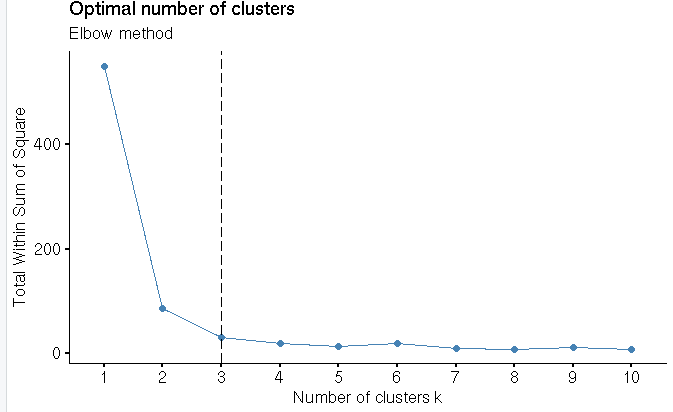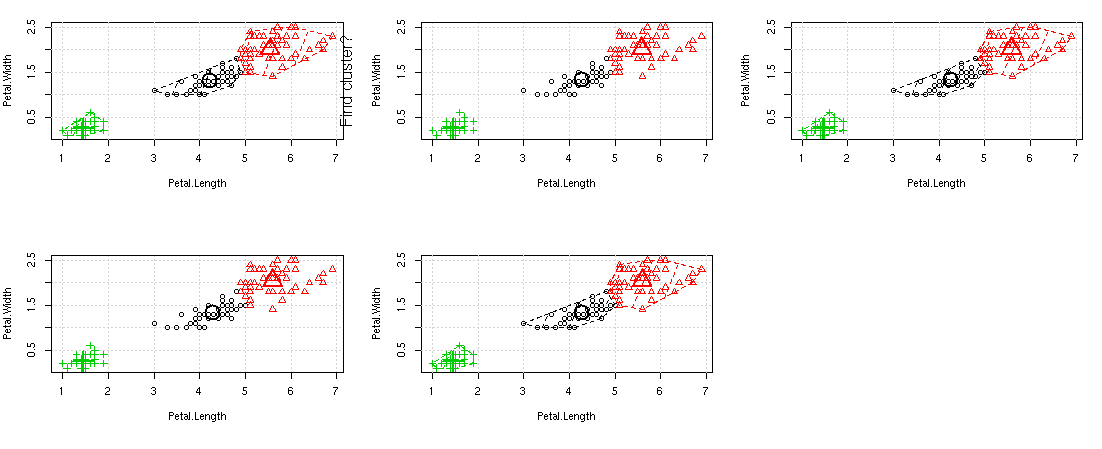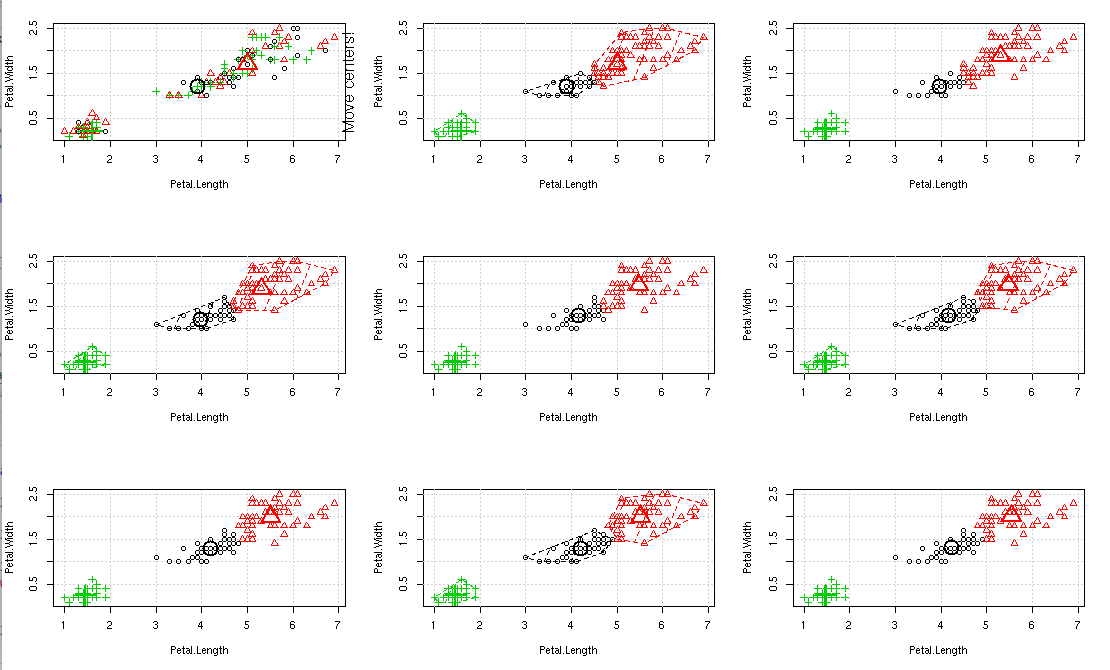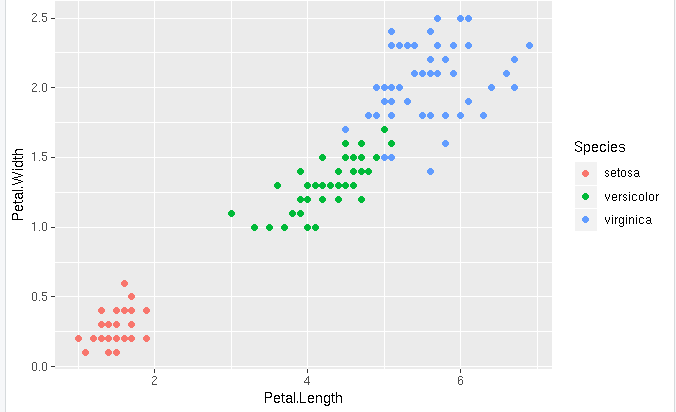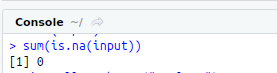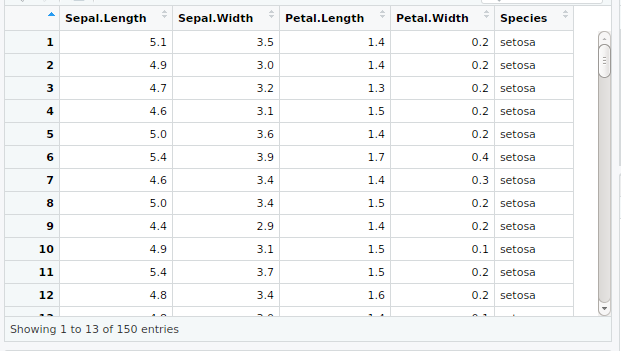• #5, First Floor, 4th Street Dr. Subbarayan Nagar Kodambakkam, Chennai-600 024 Landmark : Samiyar Madam
• pro@slogix.in
• +91- 81240 01111

### How to implement kmeans clustering for the given data in R?

###### Description

To implement the kmeans clustering for the given data set using R programming.

###### Process
What is Unsupervised ML?

In unsupervised learning all the data are unlabelled.

It have input data (x) and no corresponding output (y) variable.

Its goal is to model the underlying structure of the data and to distribute the data.

It is grouped into Clustering and Association.

Clustering:

To discover groupings in the dataset.

Centroid based Algorithms : K-means,hierarchical algorithm.

K-means Clustering algorithm:

It is a type of Unsupervised learning.

One of the clustering algorithm

It is a centroid based algorithm

To find Patterns in the data.

Steps in K-means Clustering:

Step 1: Import data.

Step 2: Data Preparation

Step 3: Compute kmeans

Step 4: Ploting the result.

Step 5: Finding the optimal number of clusters.

Package and Functions:

R Package :ggplot2--For Visualization

R Package : animation--For animatic visualization

R Package :factoextra--For data manipulation and visualization.

R Package : NbClust--For Visualizing the number of clusters.

R Function : sum(is.na(data))--to return the number of missing values.

R Function : kmeans(data,centers=,nstart= )-- to compute kmeans.

data--data set to be clustered

centers--No.of Clusters to be formed

nstart --No.of random sets to be chosen initially.

R Function : fviz_nbclust(x,FUNcluster method=c(silhoutte,wss,gap_stat))-from factoextra package used to compute three different methods(silhoutte,elbow,gap statistic) for any partitioning clustering methods(k-means,k-mediods,HCUT)

R Function : par(mfrow=c())--to split the graph screen

###### Sapmle Code

#Clustering

#Input Data set

input View(input)

#Data Preparation

#Missing Values

sum(is.na(input))

#ggplot

#install.packages(“ggplot2”)

library(“ggplot2”)

ggplot(input, aes(x=Petal.Length,y=Petal.Width,color

=Species)) +geom_point()

#kmeans

kmeans(input[,3:4],centers = 3,nstart = 25)

#install.packages(“animation”)

library(“animation”)

par(mfrow=c(3,3))

kmeans.ani(input[,3:4],3)

#Finding optimal k

km print(km)

km\$tot.withinss

#Elbow method

#install.packages(“factoextra”)

library(“factoextra”)

#install.packages(“NbClust”)

library(“NbClust”)

fviz_nbclust(input[,3:4],kmeans,method = “wss”) + geom_vline(xintercept = 3,linetype=5) + labs(subtitle=”Elbow method”)

###### Screenshots